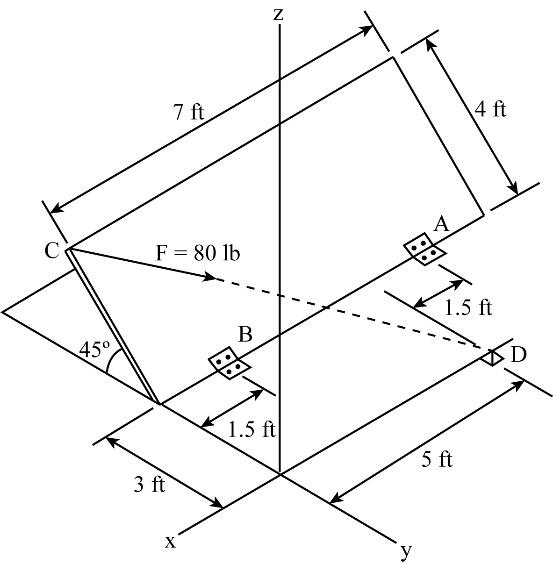×
Get Full Access to Engineering Mechanics: Statics - 14 Edition - Chapter 4 - Problem 4-39
Get Full Access to Engineering Mechanics: Statics - 14 Edition - Chapter 4 - Problem 4-39

×

# Solution: Determine the moment of the force F about the doorISBN: 9780133918922 126

## Solution for problem 4-39 Chapter 4

Engineering Mechanics: Statics | 14th Edition

• Textbook Solutions
• 2901 Step-by-step solutions solved by professors and subject experts
• Get 24/7 help from StudySoup virtual teaching assistantsEngineering Mechanics: Statics | 14th Edition

4 5 1 350 Reviews
23
4
Problem 4-39

Determine the moment of the force F about the door hinge at B. Express the result as a Cartesian vector. 5 ft 1.5 ft 1.5 ft 3 ft 7 ft 4 ft z C A B D x y F 80 lb 45 Probs. 438/39

Step-by-Step Solution:

Problem 4-39

Determine the moment of the force F about the door hinge at B. Express the result as a Cartesian vector.,,,,,,and.Step by Step Solution

Step 1 of 3

Given Data:

The force is.

The angle is.

The coordinates of point B, C and D are,andrespectively.

In order to determine the moment of force F, we need to calculate the distance among B and C.Step 2 of 3

Step 3 of 3

##### ISBN: 9780133918922

Unlock Textbook Solution